# Calculate the Net Force

## Homework Statement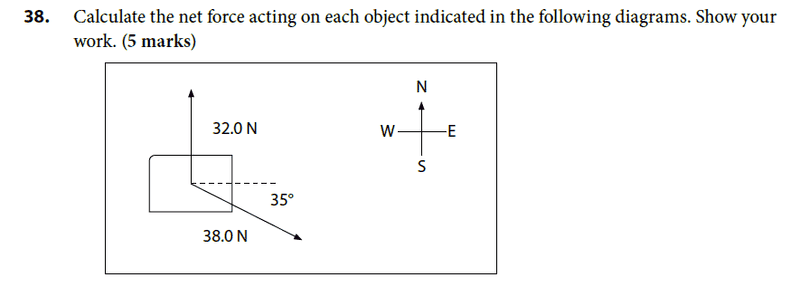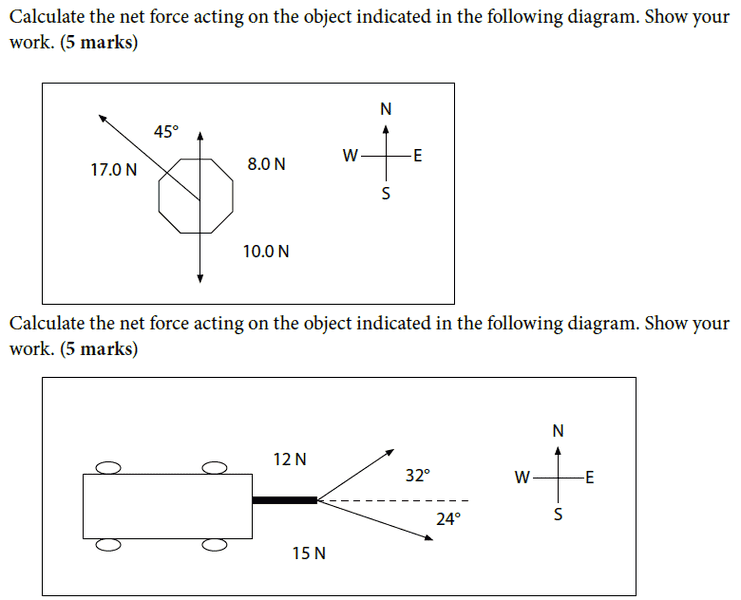## The Attempt at a Solution

38)
c^2=a^2=b^2-2abcosC
c=([32.0N]^2=[38.0N]^2-2[32N]*[38N]cos125)1/2
c=62.15N

sin law
32/sinA=62.2N/sin125
=24.8 or 25

SteamKing
Staff Emeritus
Homework Helper

## Homework Statement

View attachment 84082 View attachment 84082 View attachment 84083 View attachment 84083

## The Attempt at a Solution

38)
c^2=a^2=b^2-2abcosC
c=([32.0N]^2=[38.0N]^2-2[32N]*[38N]cos125)1/2
c=62.15N

sin law
32/sinA=62.2N/sin125
=24.8 or 25

Unless I misunderstand the problem statement, the net force here will be the algebraic sum of the two force vectors. Applying the law of cosines as you did gives you the difference of the two force vectors.

Also, you must supply a direction along with the magnitude of the force in order to have a complete answer.

As SteamKing mentioned, you've calculated the magnitude of the difference of the two vectors, not the magnitude of the net force, which you would get by a vector sum.

The point of all these problems is to resolve the forces into two perpendicular components, an x-component and y-component, by using trigonometry, in order to simplify the addition of vectors. After you've resolved all the forces into their components, you just add all the x-components together to get a net force ##F_x## in the x-direction and add all the y-components to get a net force ##F_y## in the y-direction. The total net force ##F_{net}## will be the vector sum of ##F_x## and ##F_y##, and since these two are perpendicular to each other, you just need to use the Pythagorean theorem to determine that the magnitude of ##F_{net}## will be ##\sqrt{F_x^2+F_y^2}##. To determine the direction of the net force, you need to find the angle in the triangle with sides ##F_x##, ##F_y## and ##F_{net}##, which can be determined using ##\tan{\alpha}=\frac{F_y}{F_x}##, and thus ##\alpha=\arctan{\frac{F_y}{F_x}}##.

You can find some examples at http://www.physicsclassroom.com/Class/vectors/u3l3b.cfm and http://www.physicsclassroom.com/class/vectors/Lesson-3/Net-Force-Problems-Revisited.

I left the vectors out of the equation, but i do know that i need to put them into my final answer.

As SteamKing mentioned, you've calculated the magnitude of the difference of the two vectors, not the magnitude of the net force, which you would get by a vector sum.

The point of all these problems is to resolve the forces into two perpendicular components, an x-component and y-component, by using trigonometry, in order to simplify the addition of vectors. After you've resolved all the forces into their components, you just add all the x-components together to get a net force ##F_x## in the x-direction and add all the y-components to get a net force ##F_y## in the y-direction. The total net force ##F_{net}## will be the vector sum of ##F_x## and ##F_y##, and since these two are perpendicular to each other, you just need to use the Pythagorean theorem to determine that the magnitude of ##F_{net}## will be ##\sqrt{F_x^2+F_y^2}##. To determine the direction of the net force, you need to find the angle in the triangle with sides ##F_x##, ##F_y## and ##F_{net}##, which can be determined using ##\tan{\alpha}=\frac{F_y}{F_x}##, and thus ##\alpha=\arctan{\frac{F_y}{F_x}}##.

You can find some examples at http://www.physicsclassroom.com/Class/vectors/u3l3b.cfm and http://www.physicsclassroom.com/class/vectors/Lesson-3/Net-Force-Problems-Revisited.

we haven't been shown how to break things down into components in this unit, so we wouldn't be required to use it. The only examples shown to us how to find net force is this formula: c^2=a^2+b^2 -2abcosC would this be the right formula for finding the resultant force?

Last edited:
Unless I misunderstand the problem statement, the net force here will be the algebraic sum of the two force vectors. Applying the law of cosines as you did gives you the difference of the two force vectors.

Also, you must supply a direction along with the magnitude of the force in order to have a complete answer.

i need to find the angle in order to figure out the direction. what formula do i use?

Also, can anyone help me with the other two diagrams? I uploaded them in my initial post.

SteamKing
Staff Emeritus
Homework Helper
i need to find the angle in order to figure out the direction. what formula do i use?
You've been given some other hints by Helium4. Have you tried to use those to solve this problem?

BTW, concentrate on solving this problem first. The other problems are similar, and you won't be able to tackle them successfully if you don't understand what's going on here.

You've been given some other hints by Helium4. Have you tried to use those to solve this problem?

BTW, concentrate on solving this problem first. The other problems are similar, and you won't be able to tackle them successfully if you don't understand what's going on here.
The way that it is shown to me is using sinA/A=sinB/B=sinC/C or tan=opp/adj

so what i did was 62.2N/sin125=32.0N/sin
=24.8 or 25 deg

we haven't been shown how to break things down into components in this unit, so we wouldn't be required to use it. The only examples shown to us how to find net force is this formula: c^2=a^2+b^2 -2abcosC would this be the right formula for finding the resultant force?

I'm assuming you haven't learned about force components, so a different solution to this problem might be to complete a parallelogram with sides equal to the two force vectors and use a Cosine Rule in that parallelogram (see the attached image). When you have completed such a parallelogram, you can use the Cosine Rule in the triangle with sides ##F_1##, ##F_2## and ##F_{net}##, as shown in the image, from which you can determine ##F_{net}## using $$F_{net}^2=F_1^2+F_2^2-2\cdot F_1 \cdot F_2 \cdot \cos{(180 -\alpha)}$$ Once you've done that, then you can also find the angle of ##F_{net}## using the Sine Rule in the same triangle.

So, try completing a parallelogram in your problem and see if you can apply the Cosine Rule to that parallelogram.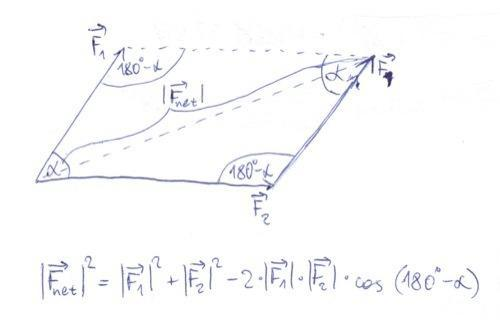#### Attachments

Last edited:
I'm assuming you haven't learned about force components, so a different solution to this problem might be to complete a parallelogram with sides equal to the two force vectors and use a Cosine Rule in that parallelogram (see the attached image). When you have completed such a parallelogram, you can use the Cosine Rule in the triangle with sides ##F_1##, ##F_2## and ##F_{net}##, as shown in the image, from which you can determine ##F_{net}## using $$F_{net}^2=F_1^2+F_2^2-2\cdot F_1 \cdot F_2 \cdot \cos{(180 -\alpha)}$$ Once you've done that, then you can also find the angle of ##F_{net}## using the Sine Rule in the same triangle.

So, try completing a parallelogram in your problem and see if you can apply the Cosine Rule to that parallelogram.

View attachment 84125
this is what i get by using the method you suggested to me:

180deg-35deg=145deg

Fnet^2= F1^2+F^2 -2(F1)(F2) cosC
Fnet^2=32N^2+38^2 -2(32N)(38N)cos145
Fnet^2= 4460.17
square root of 4460.17 = 66.78 or 67N

sin 145/67N=sin/38
sin=38N sin145/67N
sin=21.79/67N
sin-1(0.3253)
=18.9 or 19deg

Fnet=67N [N 19deg W]

is this right?

this is what i get by using the method you suggested to me:

180deg-35deg=145deg

Fnet^2= F1^2+F^2 -2(F1)(F2) cosC
Fnet^2=32N^2+38^2 -2(32N)(38N)cos145
Fnet^2= 4460.17
square root of 4460.17 = 66.78 or 67N

sin 145/67N=sin/38
sin=38N sin145/67N
sin=21.79/67N
sin-1(0.3253)
=18.9 or 19deg

Fnet=67N [N 19deg W]

is this right?

This is still incorrect because the case I described holds for a general case where ##\alpha < 90##, which isn't the case in your problem #38, but holds for example for the last problem you attached. For the case where ##\alpha > 90##, which is the case in problem #38, you would need to draw a slightly different parallelogram, but the principle is the same: you want the diagonal to be ##F_{net}## and the two sides to be the two given vectors ##F_1## and ##F_2##, from which you can determine ##F_{net}## using the Cosine Rule in that triangle. To help you a bit more, see the following drawing for problem #38, where I've calculated the angle between ##F_1=38N## and ##F_2=32N## to be 180-90-35=55 degrees. Now you should be able to calculate ##F_{net}## using Cosine Rule and its angle using the Sine Rule.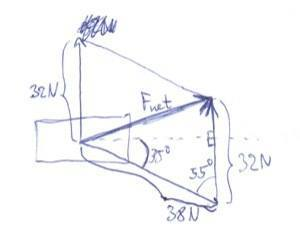Last edited:
This is still incorrect because the case I described holds for a general case where ##\alpha < 90##, which isn't the case in your problem #38, but holds for example for the last problem you attached. For the case where ##\alpha > 90##, which is the case in problem #38, you would need to draw a slightly different parallelogram, but the principle is the same: you want one side to be ##F_{net}## and the other two sides to be the two given vectors ##F_1## and ##F_2##, from which you can determine ##F_{net}## using the Cosine Rule in that triangle. To help you a bit more, see the following drawing for problem #38, where I've calculated the angle between ##F_1=38N## and ##F_2=32N## to be 180-90-35=55 degrees. Now you should be able to calculate ##F_{net}## using Cosine Rule and its angle using the Sine Rule.

View attachment 84139
I knew that the angle part couldn't be right!

so this would be my answer now that i have changed the angle to 55deg:

90deg-35=55deg

Fnet^2= F1^2+F^2 -2(F1)(F2) cosC
Fnet^2=32N^2+38^2 -2(32N)(38N)cos55
Fnet^2= 1073
square root of 1073 = 32.7N or 33N

sin 55/33N=sin/38
sin=38N sin55/33N
sin=31.12/33
sin-1(0.9432)
=70.6deg or 71 deg

Fnet= 33N [N 71deg W]

if this is correct, i think i should be able to answer the second and third question

I knew that the angle part couldn't be right!

so this would be my answer now that i have changed the angle to 55deg:

90deg-35=55deg

Fnet^2= F1^2+F^2 -2(F1)(F2) cosC
Fnet^2=32N^2+38^2 -2(32N)(38N)cos55
Fnet^2= 1073
square root of 1073 = 32.7N or 33N

sin 55/33N=sin/38
sin=38N sin55/33N
sin=31.12/33
sin-1(0.9432)
=70.6deg or 71 deg

Fnet= 33N [N 71deg W]

if this is correct, i think i should be able to answer the second and third question

Great, you're almost there. The magnitude of the net force is correct, but you calculated a different angle. If you look at the drawing, you'll see that the angle you calculated is actually the angle between the vectors ##F_{net}=33N## and ##F_2=32N##, but what you want is the angle between the vector ##F_{net}## and the horizontal. So, what would be the correct angle?

Last edited:
Great, you're almost there. The magnitude of the net force is correct, but you calculated a different angle. If you look at the drawing, you'll see that the angle you calculated is actually the angle between the vectors ##F_{net}=33N## and ##F_2=32N##, but what you want is the angle between the vector ##F_{net}## and the horizontal. So, what would be the correct angle?
angles are my weakness! would i do sin55/33=sin/32 ?

SteamKing
Staff Emeritus
Homework Helper
angles are my weakness! would i do sin55/33=sin/32 ?

You need an argument for the sin/32 bit. IOW, which angle are you talking about?

angles are my weakness! would i do sin55/33=sin/32 ?

Well, you have more options. First, you can use the angle 71° between ##F_{net}=33N## and ##F_2=32N## that you've already calculated and use the fact that the angle ##\alpha## (angle between ##F_{net}## and the horizontal), the 71° angle and a 90° angle are in the same triangle, and so you can determine ##\alpha## from that triangle.

Another option is to find the angle ##\beta## between ##F_{net}## and ##F_1=38N##, which is what you tried to do now with the Sine Rule ##\frac{sin{55}}{33}=\frac{\sin{\beta}}{32}##. For this angle, you know that ##\beta=\alpha+35°## (see the drawing), and so you can also determine ##\alpha## from there.

Also, as SteamKing pointed out, it would be nice to write the sine of angle ##\theta## as ##\sin{\theta}## instead of just ##\sin##, since we don't know what angle you're talking about.

So, what's the final angle ##\alpha##, then?

Last edited:
Well, you have more options. First, you can use the angle 71° between ##F_{net}=33N## and ##F_2=32N## that you've already calculated and use the fact that the angle ##\alpha## (angle between ##F_{net}## and the horizontal), the 71° angle and a 90° angle are in the same triangle, and so you can determine ##\alpha## from that triangle.

Another option is to find the angle ##\beta## between ##F_{net}## and ##F_3=38N##, which is what you tried to do now with the Sine Rule ##\frac{sin{55}}{33}=\frac{\sin{\beta}}{32}##. For this angle, you know that ##\beta=\alpha+35°## (see the drawing), and so you can also determine ##\alpha## from there.

Also, as SteamKing pointed out, it would be nice to write the sine of angle ##\theta## as ##\sin{\theta}## instead of just ##\sin##, since we don't know what angle you're talking about.

So, what's the final angle ##\alpha##, then?

I don't know how to write down the symbols for the angles, how do you do that?

anyways, after doing sin 55/33=sin/32 I got 52.59deg. is this right?

10N=8.0N[N]
10N-8.0=2N

Fnet^2= F1^2+F^2 -2(F1)(F2) cosC
Fnet^2=2n^2+17n^2-2*2n*17Ncos45
Fnet^2=244.9
square root of 244.9=15.6N or 16N
sin/17N=sin45/15.6
=12/15.6
sin-1(o.77)
=50deg
50deg-90deg=40deg

16N [W 40deg S]

180deg-56deg=124
Fnet^2= F1^2+F^2 -2(F1)(F2) cosC
Fnet^2=15^2=12N^2-2(15N)(12N)cos124
=24N

sin/12=sin56/24
=sin-1(0.4145)
=24.5

24N [W 24deg S]

I think we were talking about different angles. The angle between the two force vectors (55°) you calculated earlier was correct and the magnitude of the net force (33N) was correct:
90deg-35=55deg

Fnet^2= F1^2+F^2 -2(F1)(F2) cosC
Fnet^2=32N^2+38^2 -2(32N)(38N)cos55
Fnet^2= 1073
square root of 1073 = 32.7N or 33N

But the direction of the net force (i.e. the angle ##\alpha## between ##F_{net}## and the horizontal) was incorrect, because if you look at the attached image, the 71° angle you calculated is between the net force vector and the 32N force vector, not between ##F_{net}## and the horizontal:
sin 55/33N=sin/38
sin=38N sin55/33N
sin=31.12/33
sin-1(0.9432)
=70.6deg or 71 deg

What you want is the angle ##\alpha##, since that gives you the direction of ##F_{net}##. Now it should be pretty easy to calculate ##\alpha## from the image.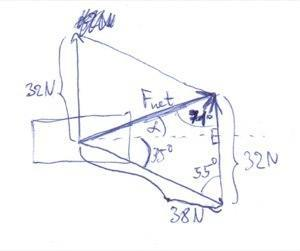I think we were talking about different angles. The angle between the two force vectors (55°) you calculated earlier was correct and the magnitude of the net force (33N) was correct:

But the direction of the net force (i.e. the angle ##\alpha## between ##F_{net}## and the horizontal) was incorrect, because if you look at the attached image, the 71° angle you calculated is between the net force vector and the 32N force vector, not between ##F_{net}## and the horizontal:

What you want is the angle ##\alpha##, since that gives you the direction of ##F_{net}##. Now it should be pretty easy to calculate ##\alpha## from the image.

View attachment 84167

is it sin 55/33=sin/32
sin-1(o.7943)
=52.59 or 53deg

you said that the B= A+35deg
so that would mean that A =B-35 deg?
53-35=18 deg

also, how do my other answers look?

Last edited:
is it sin 55/33=sin/32
sin-1(o.7943)
=52.59 or 53deg

you said that the B= A+35deg
so that would mean that A =B-35 deg?
53-35=18 deg

also, how do my other answers look?

Yes, the angle 18° is correct, so the correct answer for the first problem is ##F_{net}=33N## with ##\alpha=18##°. You could also have calculated ##\alpha## from the triangle where one of the angles is 71°, by doing which you would also get approximately 18°.

In your third answer, the magnitude of the net force ##F_{net}=24N## is correct, but the angle is incorrect because you have used the Sine Rule in some triangle that doesn't exist. I suggest you to draw a sketch for each problem with a completed parallelogram and angles properly annotated so that you can see in which triangles you can apply the Sine or Cosine Rule. Otherwise, just blindly applying a formula for the net force and angle doesn't have any purpose. To get you started, I have drawn a sketch for the third problem (where you already calculated ##F_{net}## to be 24N) with annotated angles, where you can use the Sine Rule to determine angle ##\beta## and once you know that, you can also find ##\alpha##.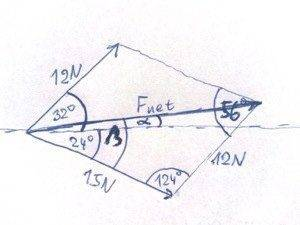The principle in the second problem is the same as in the others, but you'll need to use more parallelograms. Note that all of this would be much easier if you could just resolve all the forces into their x and y components and use the method I outlined at the beginning of this thread. Try doing some examples from the links I've posted there, it's really very easy to do.

"In your third answer, the magnitude of the net force ##F_{net}=24N## is correct, but the angle is incorrect because you have used the Sine Rule in some triangle that doesn't exist. I suggest you to draw a sketch for each problem with a completed parallelogram and angles properly annotated so that you can see in which triangles you can apply the Sine or Cosine Rule. Otherwise, just blindly applying a formula for the net force and angle doesn't have any purpose. To get you started, I have drawn a sketch for the third problem (where you already calculated ##F_{net}## to be 24N) with annotated angles, where you can use the Sine Rule to determine angle ##\beta## and once you know that, you can also find ##\alpha##.

View attachment 84182"

"The principle in the second problem is the same as in the others, but you'll need to use more parallelograms. Note that all of this would be much easier if you could just resolve all the forces into their x and y components and use the method I outlined at the beginning of this thread. Try doing some examples from the links I've posted there, it's really very easy to do."

I'm sure the answers seem simple to you, but I am having great difficulty understanding how to draw diagrams with angles, and figuring out which angle is which. Thank you for being patient with me and helping me out!

I have tried the answer once again. I think it should be:
sin124/24N=sin/15N
=31deg

31 deg-24deg =7deg

i hope this is the correct answerI have tried the answer once again. I think it should be:
sin124/24N=sin/15N
=31deg

31 deg-24deg =7deg

i hope this is the correct answerWell, this is once again incorrect because you used the Sine Rule to calculate the angle between the vectors ##F_{net}=24N## and ##12N##, which is the angle opposite to angle ##\beta## (look at the sketch). Just remember, when using the Sine Rule, you always use a pair of angle and a side opposite to that angle. Therefore, since you used the equation $$\frac{sin{124}}{24}=\frac{sin{\theta}}{15}$$ the angle 124° must be opposite to side with "length" 24N (which it is), and the angle ##\theta## which you are solving for must be opposite to side with "length" 15N. However, the angle opposite to side with "length" 15N is not ##\beta##, as you can see from the sketch. Therefore, since you want to solve for the angle ##\beta##, you would need to use the Sine Rule with side of "length" 12N instead of 15N, since the angle ##\beta## is opposite to the 12N side. So, try changing your equation for the Sine Rule to reflect that.

Well, this is once again incorrect because you used the Sine Rule to calculate the angle between the vectors ##F_{net}=24N## and ##12N##, which is the angle opposite to angle ##\beta## (look at the sketch). Just remember, when using the Sine Rule, you always use a pair of angle and a side opposite to that angle. Therefore, since you used the equation $$\frac{sin{124}}{24}=\frac{sin{\theta}}{15}$$ the angle 124° must be opposite to side with "length" 24N (which it is), and the angle ##\theta## which you are solving for must be opposite to side with "length" 15N. However, the angle opposite to side with "length" 15N is not ##\beta##, as you can see from the sketch. Therefore, since you want to solve for the angle ##\beta##, you would need to use the Sine Rule with side of "length" 12N instead of 15N, since the angle ##\beta## is opposite to the 12N side. So, try changing your equation for the Sine Rule to reflect that.

so now I've tried tried what you suggested and I got 24.48 deg. Is this right?